# Make 24

Here comes an engaging challenge for poker masters and math geniuses alike - can you compose equations which give 24 by mixing arithmetic signs and brackets with playing cards? At each round of the game you will be given 4 randomly dealt cards, as well as the arithmetic signs of addition, subtraction, multiplication and division, plus a pair of brackets. The cards carry numerical values same as their numbers, and A equals to 1, J equals to 11, Q equals to 12 and K equals to 13. Click and drag all of the cards to the blackboard underneath, then click to choose suitable arithmetic signs at the bottom left corner to compose a formula that gives 24. You may rearrange the positions of the components on the blackboard by dragging them. When you have finished the task, click the Submit button at the bottom right corner to check whether the formula is valid. If your formula is not correct, you need to rearrange the components again in order to proceed. Each round you will be given 60 seconds t

## Related software (5)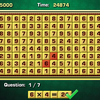## Arithmetic Game

Complete the equations in the interesting arithmetic test!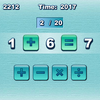## Quick Math

How quickly can you complete the equations with arithmetic symbols?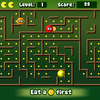## Math Man

Play Ghost Man with a mathematical twist!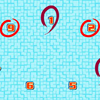## Make 15

Pick 3 numbers that add up to 15.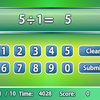## Quick Calculate

Showcase your arithmetic skills to get the answers!